# 20202315 实验九《数据结构与面向对象程序设计》实验报告

## 1.实验内容

(1) 初始化：根据屏幕提示（例如：输入1为无向图，输入2为有向图）初始化无向图和有向图（可用邻接矩阵，也可用邻接表），图需要自己定义（顶点个数、边个数，建议先在草稿纸上画出图，然后再输入顶点和边数）（2分）
(2) 图的遍历：完成有向图和无向图的遍历（深度和广度优先遍历）（4分）
(3) 完成有向图的拓扑排序，并输出拓扑排序序列或者输出该图存在环（3分）
(4) 完成无向图的最小生成树（Prim算法或Kruscal算法均可），并输出（3分）
(5) 完成有向图的单源最短路径求解（迪杰斯特拉算法）（3分）

PS：本题12分。目前没有明确指明图的顶点和连通边，如果雷同或抄袭，本次实验0分。

## 2.实验过程及结果

(1) 初始化：根据屏幕提示（例如：输入1为无向图，输入2为有向图）初始化无向图和有向图（可用邻接矩阵，也可用邻接表），图需要自己定义（顶点个数、边个数，建议先在草稿纸上画出图，然后再输入顶点和边数）

·这里我使用的是邻接矩阵实现无向图及有向图的初始化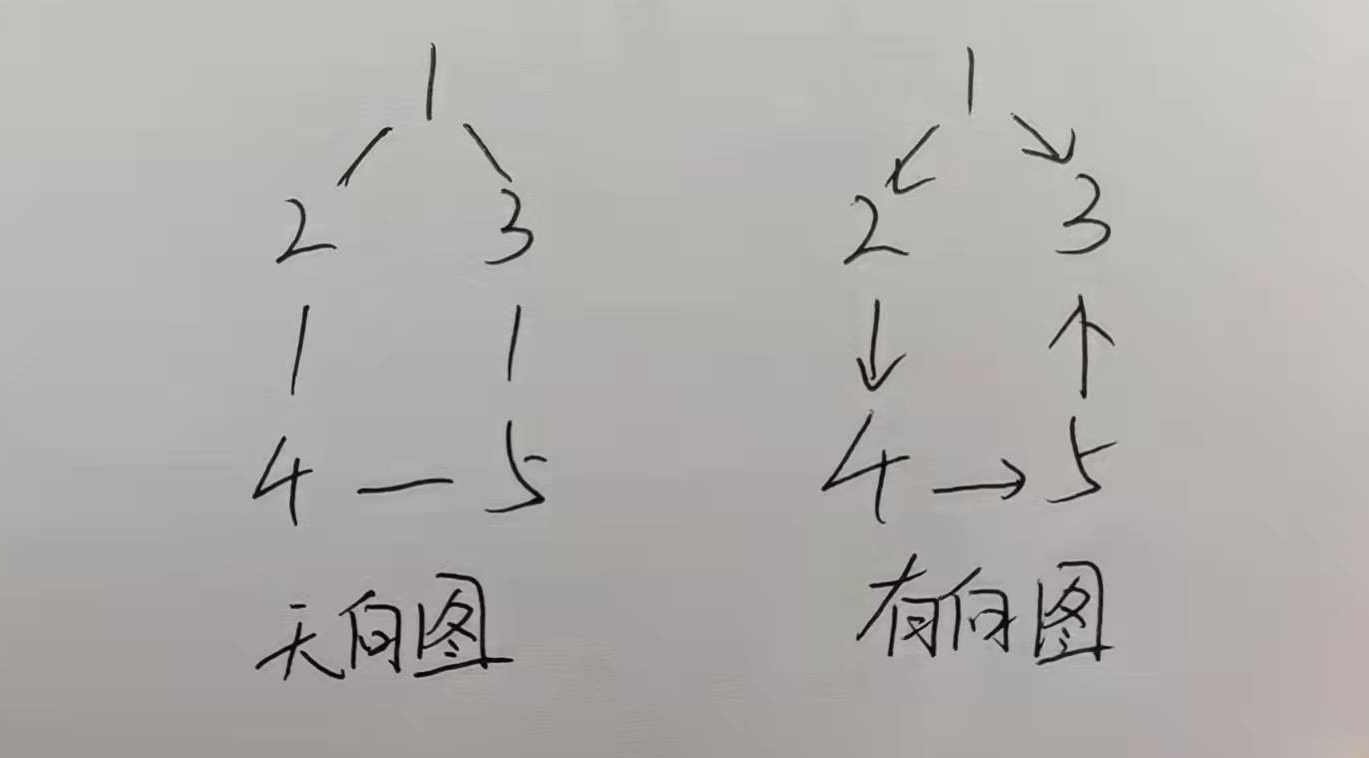运行结果截图如下：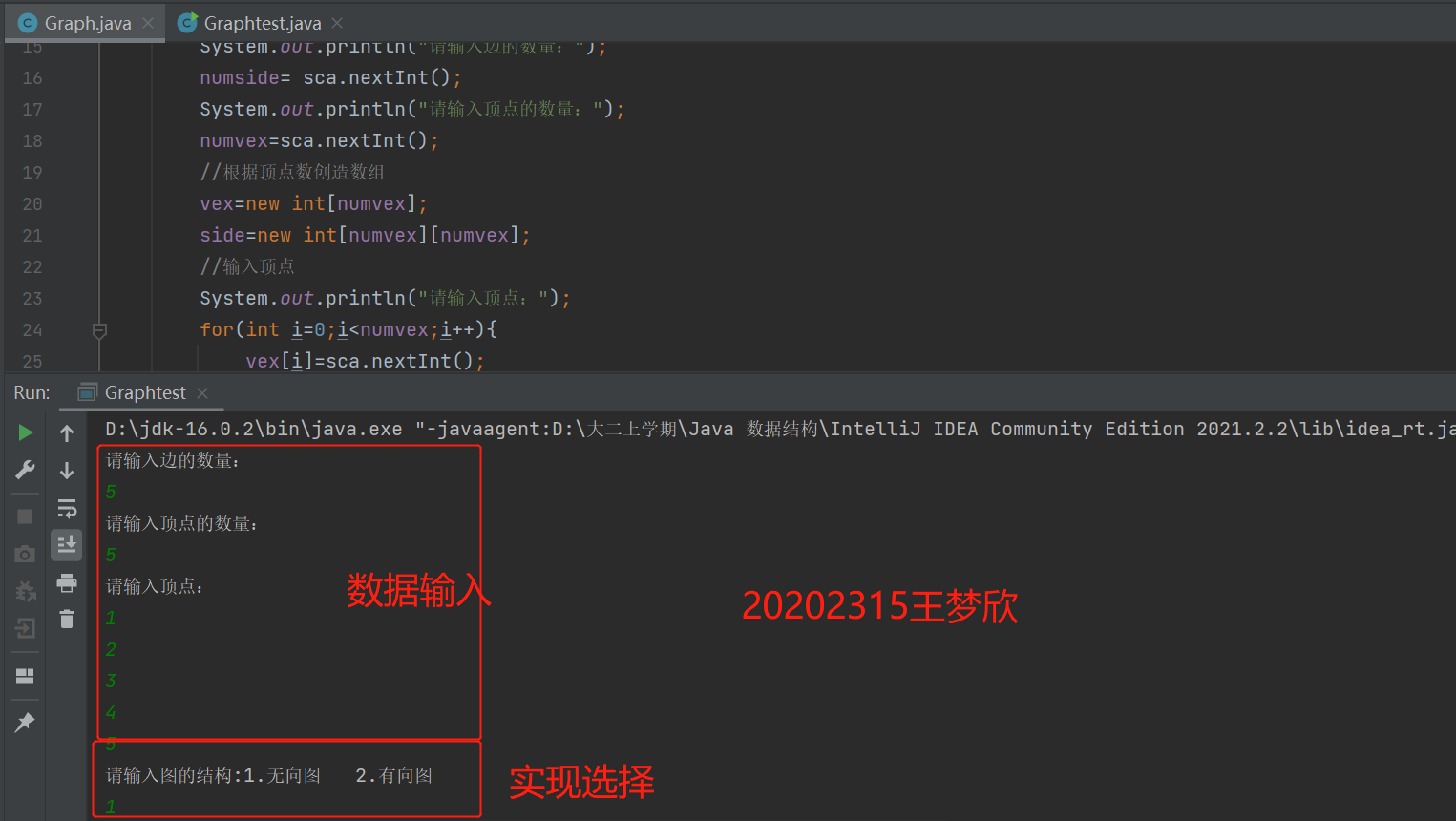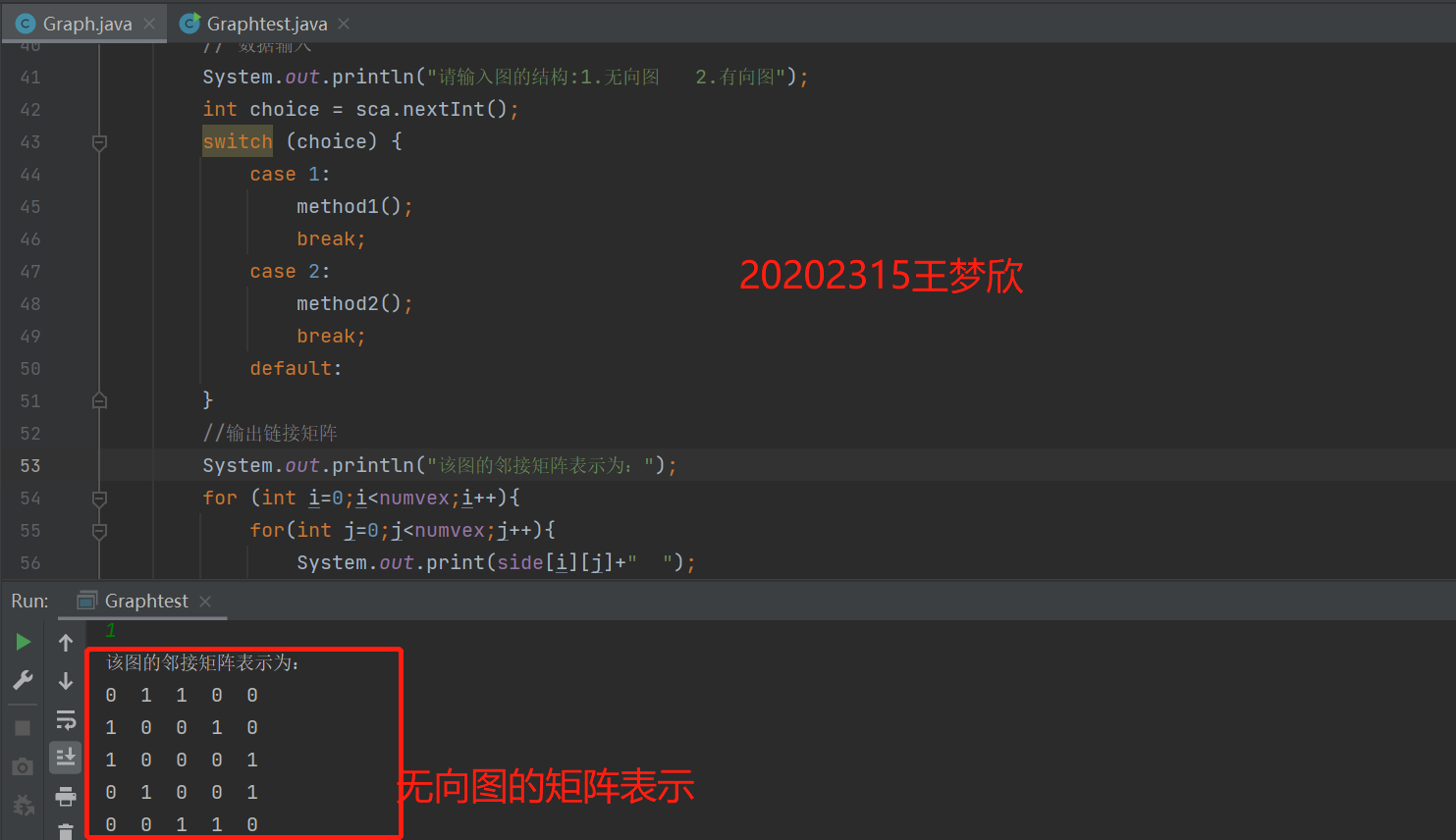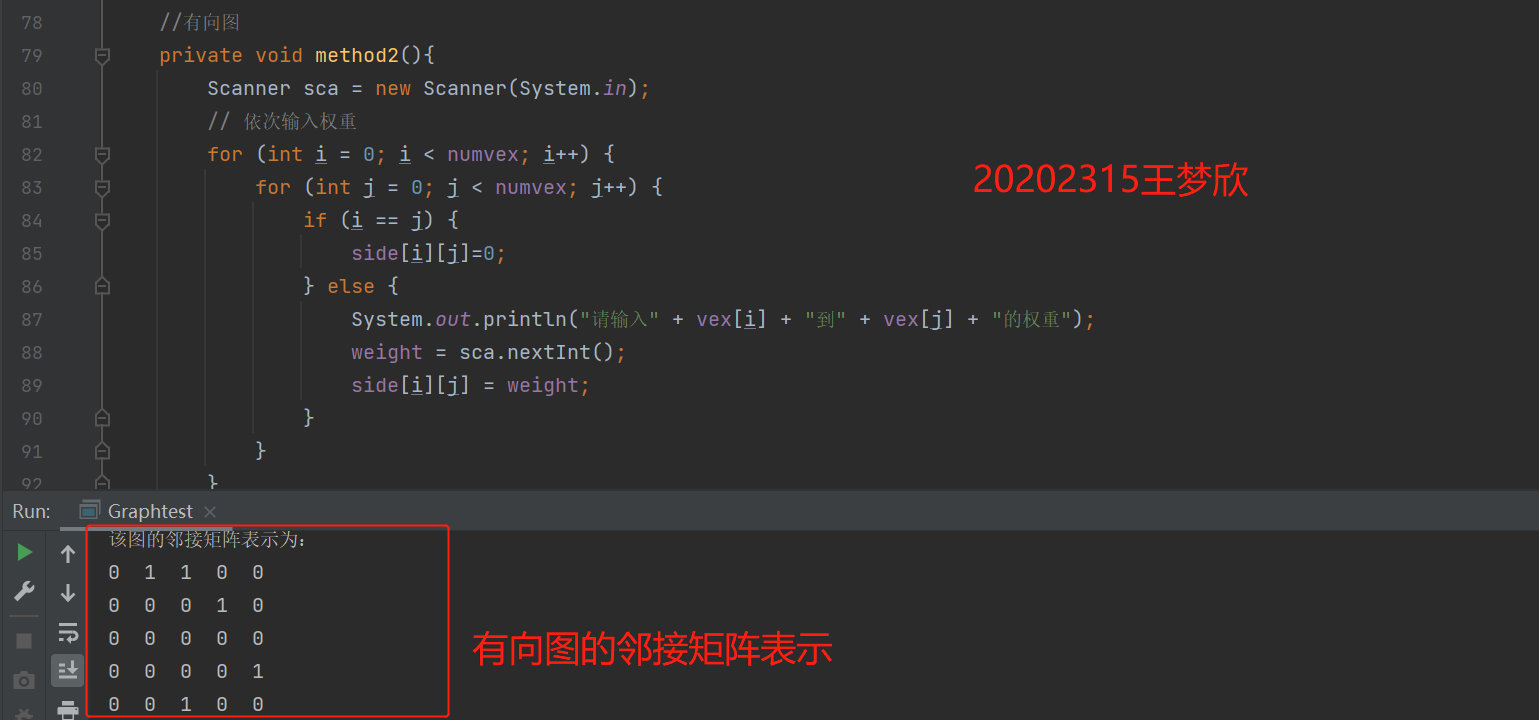(2) 图的遍历：完成有向图和无向图的遍历（深度和广度优先遍历）

//得到第一个邻接结点的下标
public int getFirstNeighbor(int index){
for (int j=0;j<numvex;j++){
if(side[index][j]>0){
return j;
}
}
return -1;
}
//根据前一个邻接结点的下标来确定下一个邻接结点
public int getNextNeighbor(int v1,int v2){
for(int j=v2+1;j<numvex;j++){
if(side[v1][j]>0){
return j;
}
}
return -1;
}
//私有函数，深度优先遍历
private void depthFirstSearch(boolean[] isVisited,int i){
//首先访问该结点，在控制台打印出来,置该结点为已访问
System.out.print(vex[i]+" ");
isVisited[i]=true;

int w=getFirstNeighbor(i);
while(w!=-1){
if(!isVisited[w]){
depthFirstSearch(isVisited,w);
}
w=getNextNeighbor(i,w);
}
}
//对外公开函数，深度优先遍历，与其同名私有函数属于方法重载
public void depthFirstSearch(){
boolean[] isVisited = new boolean;
for(int i=0;i<numvex;i++){
if(!isVisited[i]){
depthFirstSearch(isVisited,i);
}
}
}
//私有函数，广度优先遍历
int u,w;
//首先访问该结点，置该结点为已访问
System.out.print(vex[i]+" ");
isVisited[i]=true;
//结点进入队列
while (!quene.isEmpty()){
u=((Integer)quene.removeFirst()).intValue();
w=getFirstNeighbor(u);
while(w!=-1){
if(!isVisited[w]){
//访问该结点、标记并入队列
System.out.print(vex[w]+" ");
isVisited[w]=true;
}
//寻找下一个邻接结点
w=getNextNeighbor(u,w);
}
}
}
//对外公开函数，广度优先遍历
boolean[] isVisited = new boolean;
for(int i=0;i<numvex;i++){
if(!isVisited[i]){
}
}
}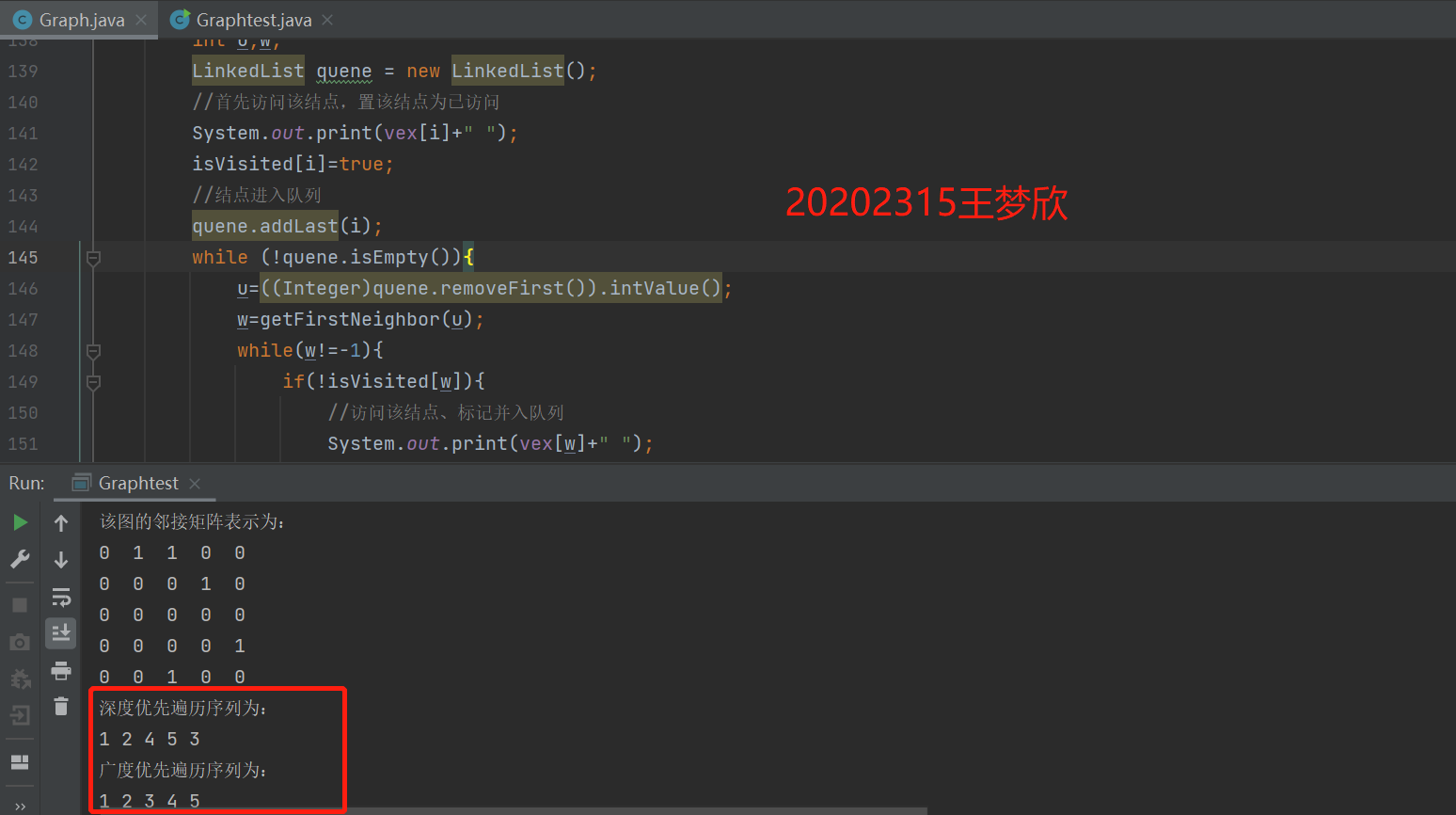(3) 完成有向图的拓扑排序，并输出拓扑排序序列或者输出该图存在环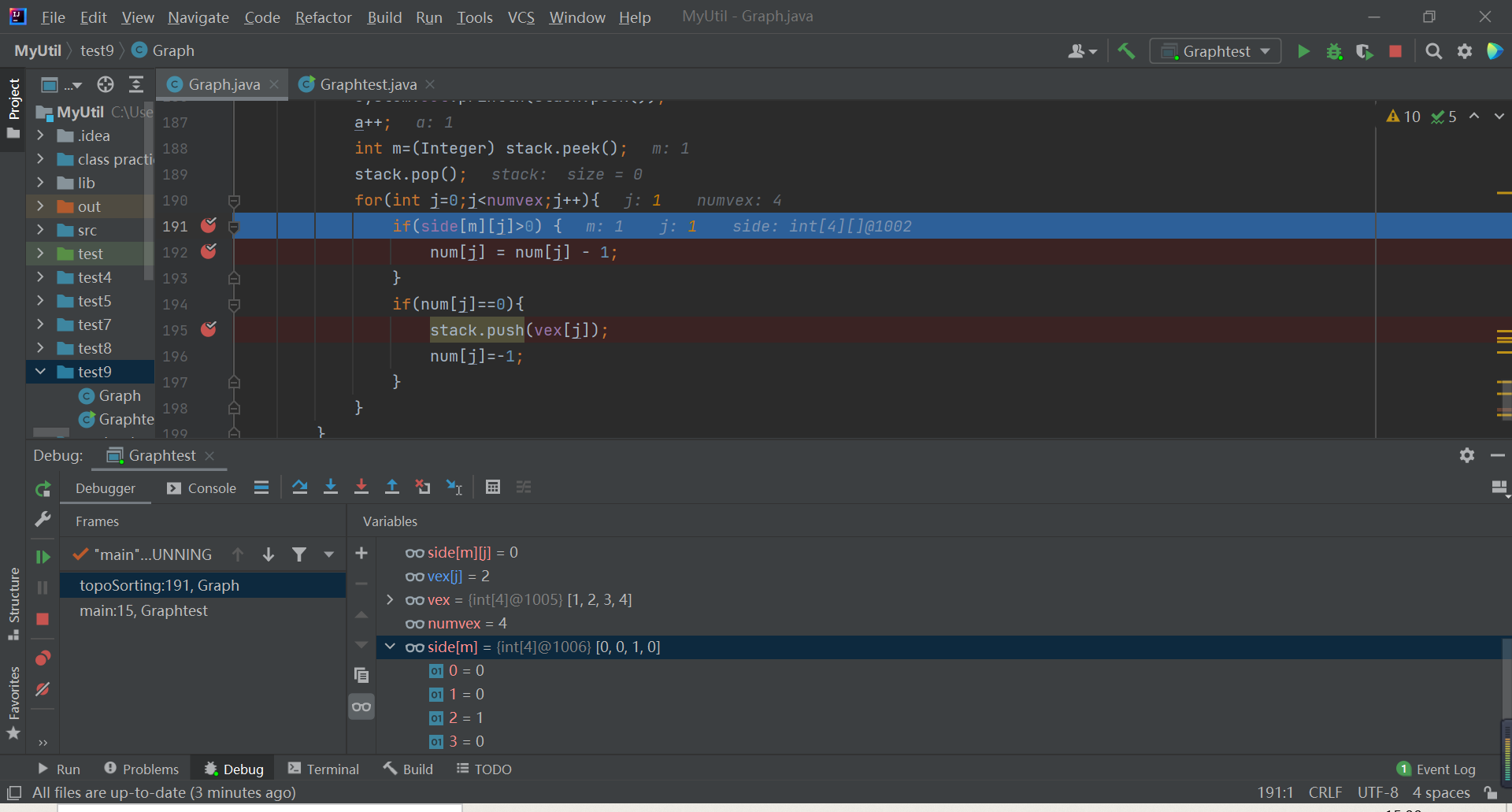//拓扑排序
public void topoSorting(){
System.out.println("拓扑排序：");
int a=0;
Stack stack = new Stack();
int[] num=new int[numvex];
for(int j=0;j<numvex;j++){
num[j] = 0;
for(int i=0;i<numvex;i++){
num[j]=num[j]+side[i][j];
}
if(num[j]==0){
stack.push(vex[j]);
num[j]=-1;
}
}
while (!stack.isEmpty()){
System.out.print(stack.peek()+"   ");
a++;
int m=(Integer) stack.peek();
stack.pop();
for(int j=0;j<numvex;j++){
if(side[m-1][j]>0) {
num[j] = num[j] - 1;
}
if(num[j]==0){
stack.push(vex[j]);
num[j]=-1;
}
}
}
if(a==numvex)
System.out.println("拓扑排序完成");
else
System.out.println("该有向图有环");
}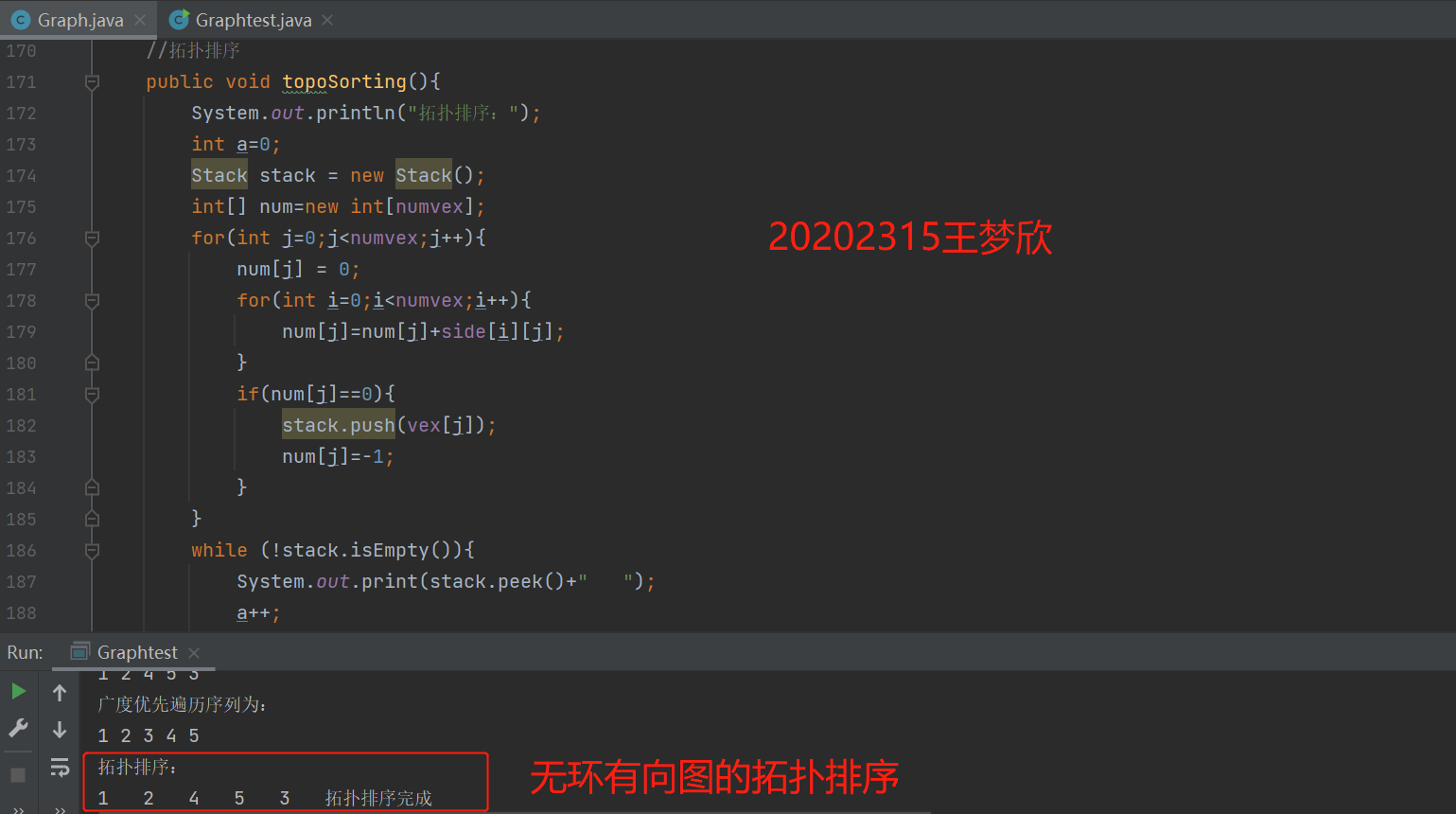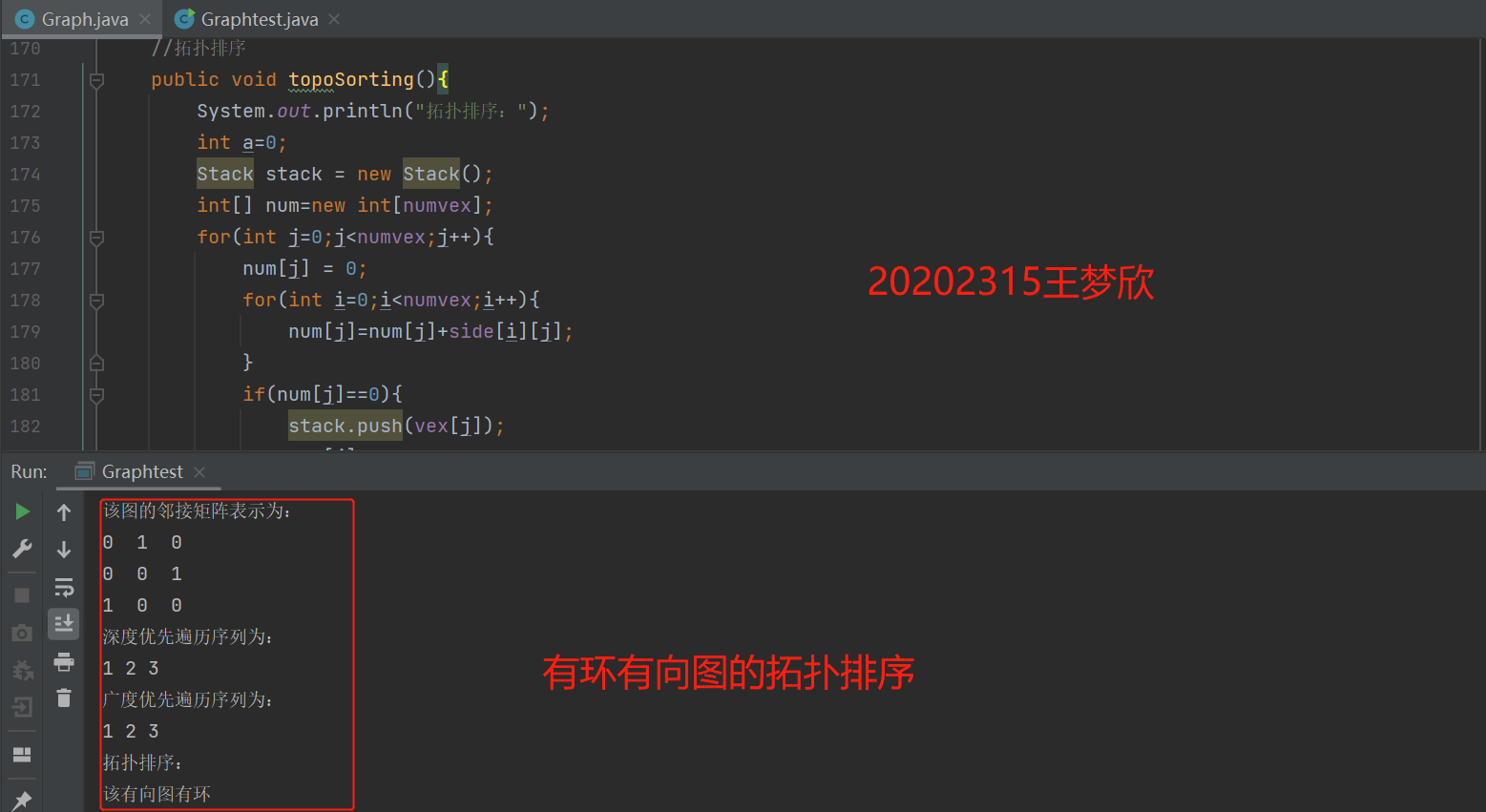(4) 完成无向图的最小生成树（Prim算法或Kruscal算法均可），并输出

·此处我采用了Prim算法，即从图中某一顶点出发, 选择与它关联的具有最小权值的边，将其顶点加入到生成树顶点集合中。重复上述步骤直到所有顶点都存在于生成树顶点集合中。

public void primCore(MGraph graph, int v) {
int visited[] = new int[graph.vertex];

visited[v] = 1;

int vertex = graph.vertex;
int minWeight = Prime.MAX;

for (int k = 1; k < vertex; k++) {

int t1 = -1;
int t2 = -1;
for (int i =0; i < vertex; i++) {
for (int j = 0; j < vertex; j++) {
boolean flag = visited[i] == 1 && visited[j] == 0 && graph.matrix[i][j] < minWeight;
if (flag) {
minWeight = graph.matrix[i][j];
t1 = i;
t2 = j;
}
}
}

//找到一条边是最小
System.out.println("边 <" + graph.data[t1] + "," + graph.data[t2] + "> 权值:" + minWeight);
visited[t2] = 1;
minWeight = Prime.MAX;
}
}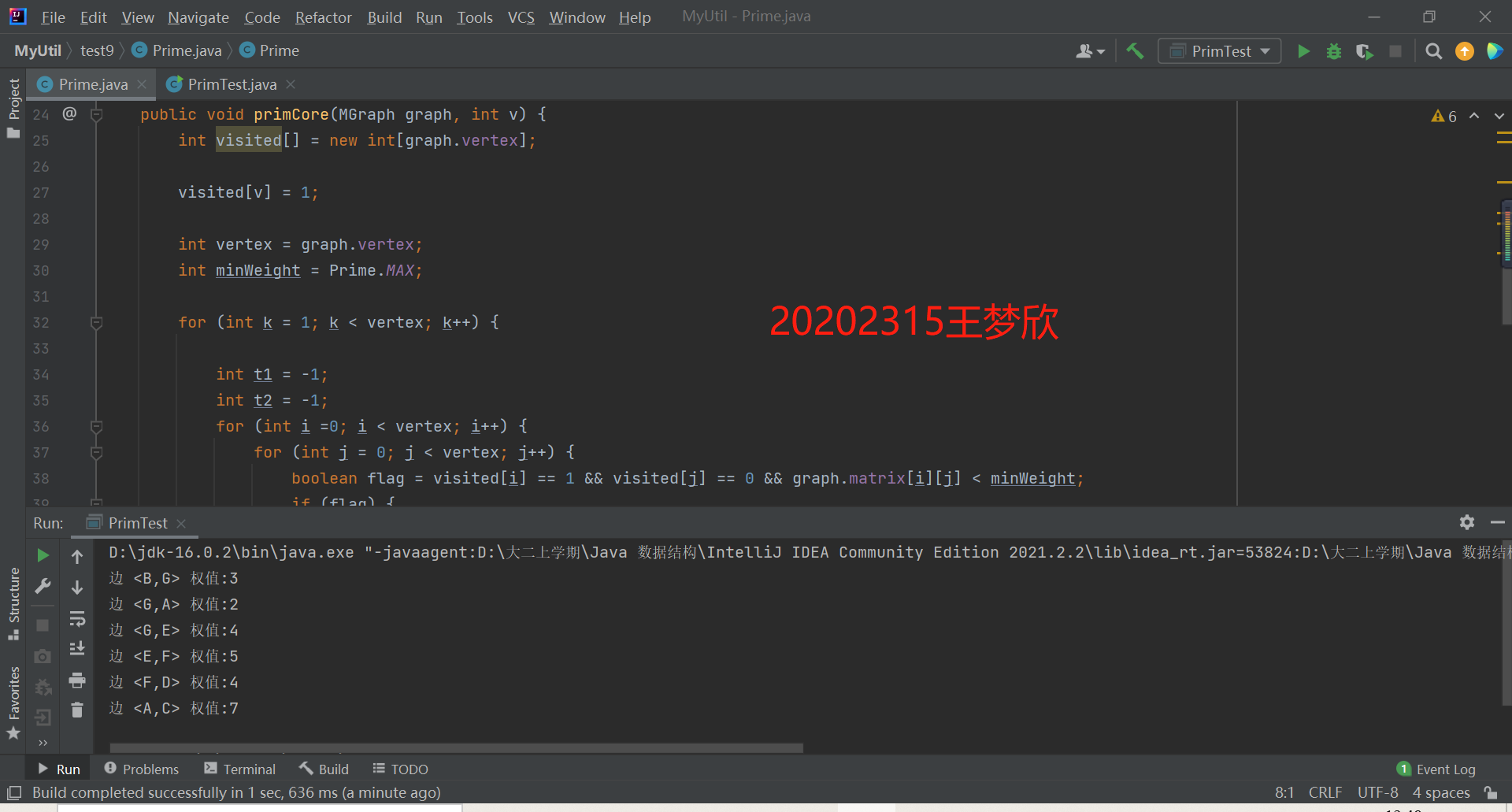(5) 完成有向图的单源最短路径求解（迪杰斯特拉算法）运行结果截图：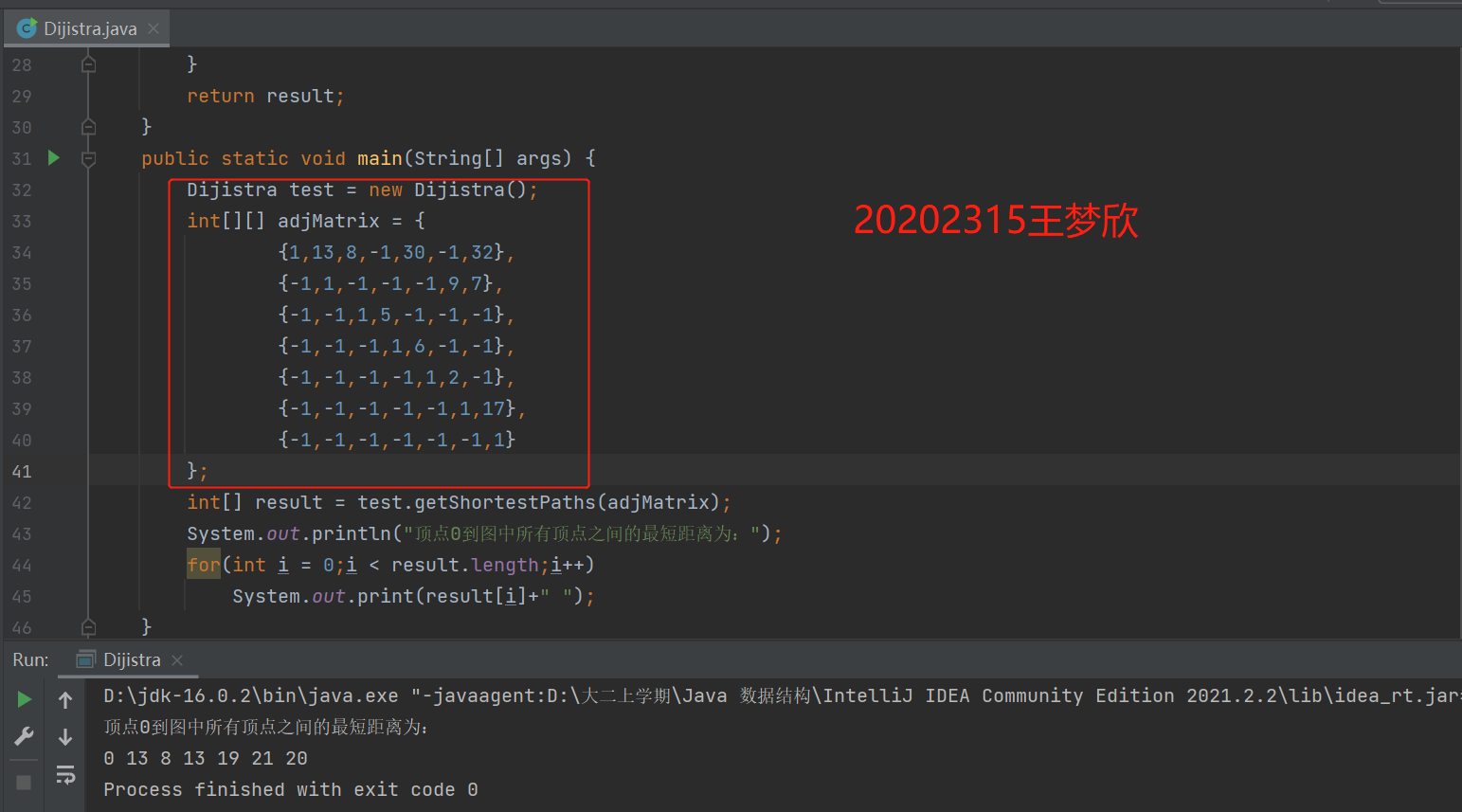## 其他（感悟、思考等）

磕磕绊绊中终于完成了最后一次实验报告，写完的这一刻心情好复杂啊，有松了一口气的轻松，也有一些惆怅，2020级是信管专业的最后一届，现在我们也走到了这门课程的最后，之前好多学长学姐和其他专业的同学说我们们很幸福，单单是遇上王老师这样的老师就已经是很幸福的一件事了，哈哈哈哈哈哈。

工作顺心

身体健康

万事胜意！

（小生以后可能还会多多叨扰恩师，望恩师见怪莫怪ღ´･ᴗ･` ）posted @ 2021-12-22 16:08  王梦欣  阅读(64)  评论(0编辑  收藏  举报